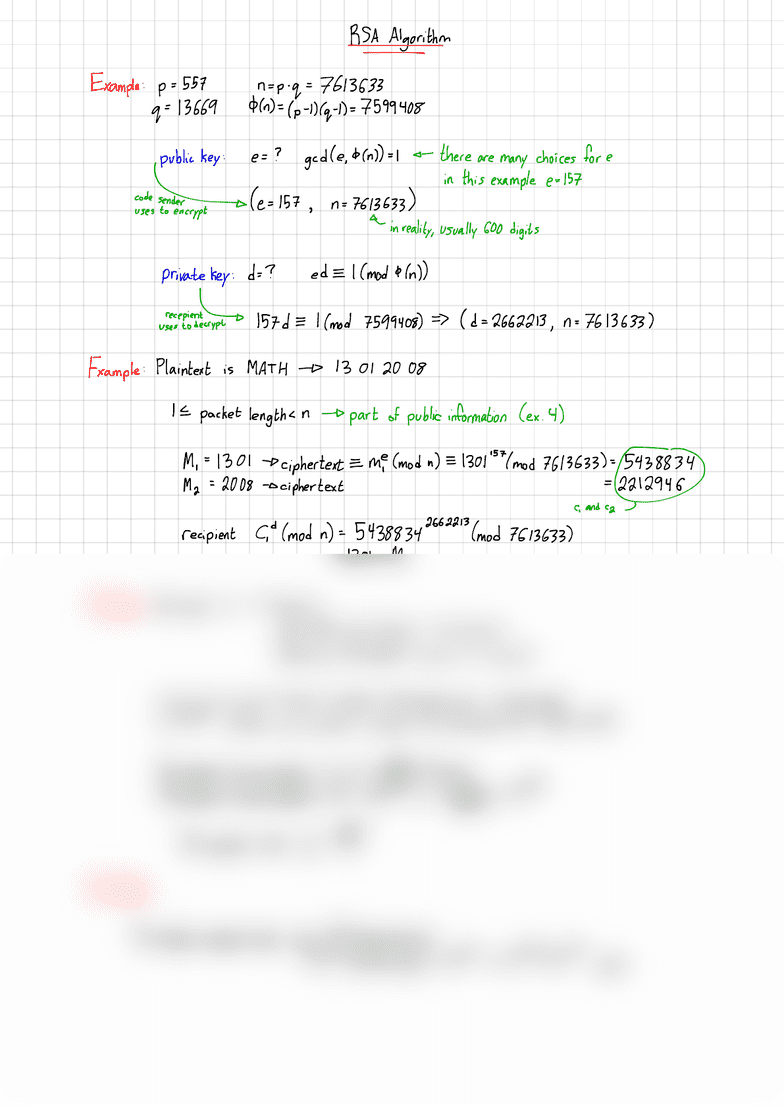Class Notes (1,100,000)
CA (620,000)
McMaster (50,000)
MATH (3,000)
Lecture 30

# MATH 1C03 Lecture Notes - Lecture 30: Plaintext

Department
Mathematics
Course Code
MATH 1C03
Professor
Miroslav Lovric
Lecture
30

This preview shows half of the first page. to view the full 2 pages of the document.Rstttttgerthm
.
Example :p=557
q= 1366g
"=P '9=7613633
\$(n)= (pt )(q-D= 7599408
public key :e=?god (e,loan ))=L there are many choices for e
in this example e- 157
a;Yeeo¥nE°(e=l57
'"
761£63
,¥d;µ,usually 600 digits
private key :D= ? ed ±1(mod ¢1n))
recepienlo lgzd =1(mod 7599408)(D= 2662213 ,n= 7613633 )
Uses to decrypt
Example :Plaintext is MATH 13 01 2008
It packet length <npart of public information (ex .4)
M,
=1301 cipher text =Me (mod n)±1301 '54mod 7613633 )=5438834
Ma =2008 cipher text =22129460
C,and cz J
recipient Gd (mod D= 543883426622 "(mod 7613633 )
=1301 =M,
To Break :We need d- need e
need &(n )=(p-l)(q.l)(not known)
need p,qfor which n=p .q(nis known)
n=p .q-op ,of are large (in reality 7300 digits each ,n=600 digits)
nt 10600 to find p.qwe need to divide nby all primes EA(At 10300)
The number of prime numbers Ex is =E) (Gauss )
The number of prime numbers em =10030 is xLemont 10297
The expected value ='s =1051
In Reality :
The fastest computer today does 1012 divisionslsecond
4.1019 divisions Gear =1020 10297/10277 years
###### You're Reading a Preview

Unlock to view full version